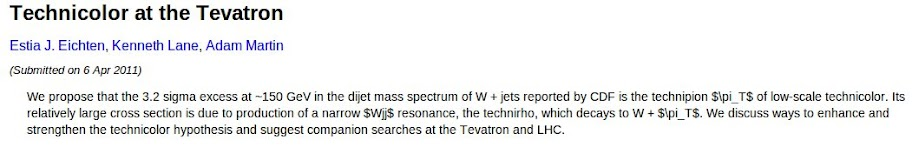### MathJax: script for LaTeX

One of the most important tool for a science blog in mathemathics and physics is the equation editor. To embed equation in post we can use a lot of solutions: for example we can write eq. on Wikipedia, past image url or download it and use in our post; we can use also LaTeX editor by codecogs.com, and also we can copy img code or download our equation.
But there's a most elegant solution: MathJax.
Using this script equations present a better integration with html pages, and using right click, our readers can read LeTeX or MathML code. For example, with Schrodinger's equation for a particle subjected by a potential $V(\vec r)$:
$i \hbar \frac{\partial}{\partial t} \psi (\vec r, t) = - \frac{\hbar}{2m} \nabla^2 \psi (\vec r, t) + V(\vec r) \psi (\vec r,t)$
An other example is the perfect integration in text. For example the abstract of the preprint Technicolor at the Tevatron:That became with MathJax:
We propose that the 3.2 sigma excess at ~150 GeV in the dijet mass spectrum of W + jets reported by CDF is the technipion $\pi_T$ of low-scale technicolor. Its relatively large cross section is due to production of a narrow $Wjj$ resonance, the technirho, which decays to W + $\pi_T$. We discuss ways to enhance and strengthen the technicolor hypothesis and suggest companion searches at the Tevatron and LHC.
There's only one controindication: the feed rss. In feed, indeed, we sent LaTeX code and not the image version.

However, to add the scrpit, in a blog on Blogspot for example, you must write the following code after <head> tag:
 <script type='text/x-mathjax-config'>       MathJax.Hub.Config({   tex2jax: {     inlineMath: [[&#39;$&#39;,&#39;$&#39;], [&#39;\$&#39;,&#39;\$&#39;]],     processEscapes: true   } }); </script>     <script type='text/x-mathjax-config'> MathJax.Hub.Config({   MMLorHTML: {     prefer: {Firefox: &quot;HTML&quot;}   } }); </script> <script src='http://cdn.mathjax.org/mathjax/1.1-latest/MathJax.js?config=TeX-AMS-MML_HTMLorMML-full' type='text/javascript'/>

I hope that the tools could be useful to other bloggers.